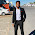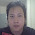## I saw a comment on my video that he wants a high gain audio amplifier (1000 times or 60 dB voltage gain!!!). However, the most commercial power amplifier IC has maximum fix gain around 200 times (lm386) that far from 1000 times voltage gain and the problem when you make high gain amplifier is the high-frequency roll-off problem.

The solution to the problem is making pre-amplifier that has very low distortion, wide bandwidth and it can amplify audio signal around 10 times or 20 dBs and send the audio signal to a power amplifier (the power amp IC he uses is tda7052 that has 40 dB or 100 times voltage gain). When combining voltage gain of the two amplifiers, he will get 1000 times voltage gain audio amplifier!!!

The method is easy and uses minimum electronic componentsBreadboard wiring
Audio preamplifier circuit diagram.

Electronics component list:IC 1 = NE5534(op amp ic)

R1,R2,C3 are amplifier gain control by negative feedback, the formula is Gain = 1 + (R2/R1)

R1 = 1 kilo-ohms

R2 = 10 kilo-ohms
C3 = 47 uf/16 volts

R3,R4,R5,C4 are opamp input voltage bias. R4 and R5 divide voltage to 1/2 power supply. C4 use to filter hum from a power supply if you use a transformer as a power supply

R3 = 10 kilo-ohms (same value as R2 is fine)

R4,R5 = 4.7 kilo-ohms
C4 = 100 uf/16 volts

C1,C2 are DC blocking capacitor

C1,C2 = 2.2 uf/16 volts

This circuit uses a single power supply so it is good for battery operation (voltage less than 12 volts)

The minimum voltage for stable operation is 5 volts so this pre-amplifier can operate by USB port as a power supply.
An input and an output signal use same ground and must use dc blocking capacitor.

If you use transformer power supply instead of a battery, you must as DC smoothing capacitor between Vcc(+) and ground, the minimum value of the cap is 470 uf/16 volts.

The video below shows you step by step guide and test.

If you have any question, feel free to ask in the comment section below.

Enjoy hobby world!!! Easyandworkproject.xyz

1.Out of all your circuits,you still havent designed an Audio amplifier(20Hz-20kHz) that shows calculations for a gain of more than 20dB and Signal distortion of less than 10%

1.The ne5534 @ gain 100 (40 dB) produce lower than 10 % THD already. Change R1 to 100 ohms and C3 to 470 microfarads.
Formula : Gain = 1 +(R2/R1)

Remember, this is only pre-amp part, combine with commercial power amp IC the gain may increase up to 10,000 time and very sensitive to radio signal and environment electromagnetic field, that is the reason I don't make the audio amplifier gain higher than 20 dB.

However, I plan to make low power (1-10 watts range) discrete audio amplifier with mosfet or transistor, stay tune.

2.How can i calculate signal distortion for a circuit?

1.Find it here.
http://www.ni.com/white-paper/3401/en/